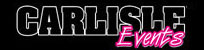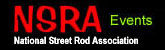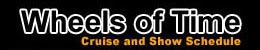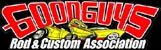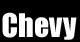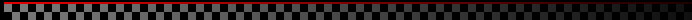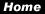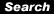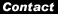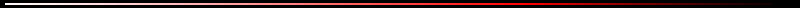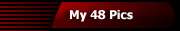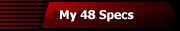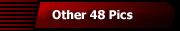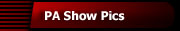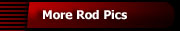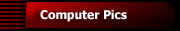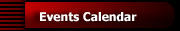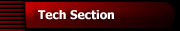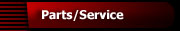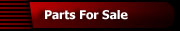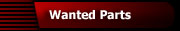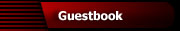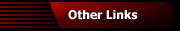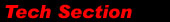Refer to the chart below to determine the diameter of a tire. Tire Size Chart (15" Wheels) Aspect Ratio Formula (Width x Aspect Ratio x 2) ÷ 25.4 + 15 Example:  205/60R15 (205 x .60 x 2) ÷ 25.4 + 15 = 24.69
 Width of tire in millimeters 35 40 45 50 55 60 65 70 75 80 Width of tire in inches

 To convert from millimeters to inches, divide by 25.4. Tire Diameter Download Excel Chart